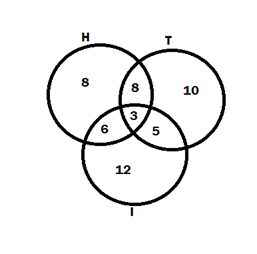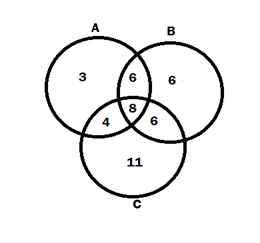# Solutions of Miscellaneous Exercise

1. Decide among the following sets, which sets are subset of one and another:

A { x : x ? R and x satisfy x2 – 8x + 12 = 0}

x2 – 8x + 12 = 0

x2 – 6x – 2x + 12 = 0

x(x – 6) - 2(x – 6) = 0

(x – 6) (x – 2) = 0

X = 6, 2

∴ A = 6, 2

B = {2, 4, 6}, C = {2, 4, 6, 8…..}, D = {6}

A ⊂ B

A ⊂ C

B ⊂ C

D ⊂ A

D ⊂ B

D ⊂ C

2. (i) if x ? A and A ? B, then, x ? B

Let A = {1, 2}

B = {3, 4, {1, 2}}

A ? B

{1, 2 } ? B

1 ∉ B, 2 ∉ B

x ∉ B

∴ False

(ii) if A ⊂ B and B ? C then, A ? C

x ? A, Let A = {1}

B = {1, 2}

C = {{1, 2}, 3}

A ∉ C

∴ False

(iii) If A ⊂ B and B ⊂ C then, A ⊂ C

x ? A              x ? C

A ⊂ B             A ⊂ C

? x ? B

B ⊂ C

∴ True

(iv) If A ⊄ B and B ⊄ C, then A ⊄ C

Let A = {1, 2}

B = {1, 3}

C = {1, 2, 4}

∴ False

(v) If x ?  A and A ⊄ B, then x ? C

Let A = {1}

B = {1, 3},

∴ False

(vi) If A ⊂ B and x ∉ B, then x ∉ A

x ? A              x ∉ B

A ? B             A ⊂ B

x ? B              x ∉ A

∴ True

3. Let A, B and C be the sets such that A B = A C and A ∩ B = A ∩ C Show that B = C.

A ∪ B = A ∪ B   ? (1)

A ∩ B) = A ∩ B   ? (2)

Show that:- B = C.

Sol:-

Taking L.H.S

B = B ∩ (A ∪ B)

= B ∩ (A ∪ C) from (1)

= (B ∩ A) ∪ (B ∩ C)

= (A ∩ C) ∪ (B ∩ C) from (2)

= (C ∩ A) ∪ (C ∩ B)

= C ∩ (A ∪ B)

= C ∩ (A ∪ C) from (1)

∴ B = C

Hence proved

4. Show that the following four conditions are equivalent.

(i) A ⊂ B

(i) ? (ii) All elements of A is in B then, A – B = ????

(ii) A – B = ????

(ii) ? (iii) All elements of A is in B then, A ∪ B = B

(iii) A ∪ B = B

(iii) ? (iv) All elements of A is in B then, A ∩ B = A

(iv) A ∩ B = A

(iv) ? (i) All elements of A is in B then, A ⊂ B

5. Show that if A ⊂ B, then C – B ⊂ C – A

Let x ? C - A

x ? C and x ∉ B

x ? C and x ∉ A   [? A ⊂ B]

x ? C – A

then, C – B ⊂ C – A

Hence proved

6. Assume that P(A) = P(B). Prove that A = B.

P(A) = P(B)       ?(1)

Show that:- A = B

Sol:-

 x ? A X ? B {x} ⊂ A {x) ⊂ B {x} ? P(A) {x} ? P(B) {x} ? P(B) from (1) {x} ? P(A) from (1) {x} ⊂ B {x} ⊂ A x ? B x ? A A ⊂ B B ⊂ A

∴ A = B

Hence proved

7. Is it true, that for any sets A and B, P(A) P(B) = P(A ∪ B)? Justify your answer

P(A) ∪ P(B) = P(A ∪ B) (given)

Let A = {1}

P(A) = { ????, {1} }

Let B = {2}

P(B) = { ????, {2}}

P(A) ∪ P(B) = {????, {1}, {2}}      -(1)

Let A ∪ B = {1, 2}

P(A ∪ B) = {????, {1}, {2}, {1, 2}}

∴ P(A) ∪ P(B) ≠ P(A ∪ B)

8. Show that you any sets A and B, A = (A ∩ B) ∪ (A – B) and A ∪ (B – A) = A ∪ B.

A = (A ∩ B) ∪ (A – B) and A ∪ (B – A) = A ∪ B

Taking R.H.S                       Taking L.H.S

(A ∩ B) ∪ (A ∩ B’)           A ∪ (B ∩ A’)

A ∩ (B ∪ B’)                                    (A ∪ B) ∩ (A ∪ A’)

A ∩ ?                                     (A ∪ B) ∩ ?

A                                             A ∪ B

Hence proved

9. using properties of sets, show that

(i) A ∪ (A ∩ B) = A

Taking L.H.S

(A ∪ A) ∩ A ∪ B)

A ∩ (A ∪ B)

= A    Hence proved.

(ii)  A ∩ (A ∪ B) = A

Taking L.H.S

(A ∩ A) ∪ (A ∩ B)

A ∪ (A ∩ B)

= A

Hence proved

10. Show that A ∩ B = A ∩ C need not imply B = C

Let A = {1, 2, 3}

B = {3, 4, 5}

C = {3, 5, 6}

A ∩ B = A ∩ C need not imply B = C (given)

{3} = {3} ? B ≠ C

Hence proved

11. Let A and B be sets. If A ∩ X = B ∩ X = ???? and A ∪ X = B ∪ X for some set X, prove that A = B

given:- A ∩ X = B ∩ X = ????     -(1)

A ∪ X = B ∪ X                            -(2)

to prove:-   A = B

Proof:- Now,

Let A = A ∪ ????

= A ∪ (B ∩ X) from (1)

= (A ∪ B) ∩ (A ∪ X)

= (B ∪ A) ∩ (B ∪ X) from (2)

= B ∪ (A ∩ X)

= B ∪ ???? from (1)

A = B

∴ A = B

Hence proved

12. find sets A, B and C such that A ∩ B, A ∩ C and B ∩ C are non-empty sets and A ∩ B ∩ C = ????

Let A = {1, 2}         A ∩ B = {2} ≠ ????

B = {2, 3}                 B ∩ C = {3} ≠ ????

C = {1, 3}                 A ∩ C = {1} ≠ ????

∴ A ∩ B ∩ C = ????

13. In a survey of 600 students in a school, 150 students were found to be drinking tea and 225 drinking coffee, 100 were drinking both tea and coffee. Find how many students were drinking neither tea nor coffee

? = 600, n(t) = 150, n(C) = 225, n(T ∩ C)

By using formula,

n(T ∪ C) = n(T) + n(C) – n(T ∩ C)

n(T ∪ C) = 150 + 225 - 100

n(T ∪ C) = 175

No. of students who take neither tea nor coffee = 600 – 275 = 325

14. In a group of students, 100 students know Hindi, 50 know English and 25 know both each of the students knows either Hindi or English. How many students are in the group.

n(H) = 100, n(E) = 150, n(H ∩ E) = 25

By using formula,

owH n(H ∪ E) = n(H) + n(E) – n(H ∩ E)

n(H ∪ E) = 100 + 50 - 25

n(H ∪ E) = 125

∴ There are 125 students who knows either Hindi or English.

15. In a survey of 60 people, it was found that 25 people read newspaper H, 26 read newspaper T, 26 newspaper I, 9 read both H & I, 11 read both H & T, 8 read both T and I, 3 read all three newspapers. Find

(i) The no. of people who read at least one of the newspaper.

(ii) The no. of people who read exactly one newspaper

N(H) = 25, n(T) = 26, n(I) = 26, n(H ∩ I) = 9, n(H ∩ I) = 11, n(T ∩ I) = 8, n(H ∩ T ∩ I) = 3

At least 1 of the newspaper = 8 + 8 + 10 + 6 + 3 + 5 + 12 = 52

Exactly 1 newspaper = 8 + 10 + 12 = 3016. In a survey it was found that 21 people liked product A, 26 liked product B, and 29 liked product C. If 14 people liked products B and A, 12 liked product C and A, 14 people liked products B & C and 8 liked all the three products. Find how many liked product C only.

Liked             product        A                     =         21Liked             product        B                     =         26

Liked             product        C                     =         29

Liked            product        A + B             =         14

Liked            product        A + C             =         12

Liked            product        B + C             =         14

Liked            product        A + B + C    =         8

∴ 11 people liked product C.

Related Keywords